# Making Sense of Positives and Negatives

##### Age 11 to 14

Published 2013

Perhaps you have heard people say "Two minuses make a plus". This isn't the most helpful way to think about positive and negative numbers.

Below are some different ways of thinking about adding and subtracting positive and negative numbers. We hope they will help you to understand what's going on when you might be tempted to use a rule like "Two minuses make a plus".

There are four possibilities that we need to be able to understand:
Adding a positive number
Adding a negative number
Subtracting a positive number
Subtracting a negative number

Hot Air Balloon
The first model we offer is the hot air balloon, as seen in the game Up, Down, Flying Around.
In this model, we represent positive numbers as 'puffs' of hot air, and negative numbers as sandbags.

 Model Calculation Outcome Adding puffs of hot air Adding a positive number Increase (in height) Adding sandbags Adding a negative number Decrease  (in height) Subtracting puffs of hot air Subtracting a positive number Decrease  (in height) Subtracting sandbags Subtracting a negative number Increase  (in height)

We can now describe a calculation such as 4 + (-2) - (+5) - (-1) + (+7) in the following way:

My balloon starts at height +4. I add two sandbags (down two), subtract five puffs of hot air (down five), subtract one sandbag (up one), then add seven puffs of hot air (up seven). My balloon ends up at height +5.

You can read the calculation as "Four add negative two, subtract positive five, subtract negative one, add positive seven", and think to yourself "Four, down two, down five, up one, up seven" or equivalent.

Happiness Model
Here is a teacher's description of how she explains positive and negative numbers to her classes:
"I believe that adding and subtracting with negative numbers makes sense.

I have a big number line ($^-10$ to $10$, say) above or along the top of my whiteboard. With the students, we brainstorm on things that are POSITIVE and things that are NEGATIVE. We talk about how you feel if someone gives you a positive thing, or if someone takes one away. We talk about how you feel if someone gives you a negative thing, or if someone takes one away.

I feel OK today, maybe I score $2$ (pointing to number line) on this happiness scale.
How would I feel if someone gave me $4$ chocolates (a generic positive!)? Yes, I move up $4$ to $6$.
Now how would it be if someone gave me a detention (negative)? Yes, down $1$, to $5$.
What if you took away $7$ of my chocolates? How would I feel? Sadder? Yes, I need to go down $7$, to $^-2$.
What if you let me off my $3$ detentions?...

Adding something positive or taking away something negative improves the situation (up the number line we go).
Adding something negative or taking away something positive makes the situation worse (down the number line we go)."

Football Model
In this model, we represent positive numbers as good footballers who score lots of goals, and negative numbers as bad footballers who score own goals. When it's time for transfers, we can add new players to our team, or take players off the team.

 Model Calculation Outcome Buy good players Adding a positive number Increase (in league position) Buy bad players Adding a negative number Decrease  (in league position) Sell good players Subtracting a positive number Decrease  (in league position) Sell bad players Subtracting a negative number Increase  (in league position)

So imagine we bought 5 good players, sold 2 good players, bought 3 bad players and sold 7 bad players. We can write the following calculation to find out the overall effect:
$+(^+5) - (^+2) + (^-3) - (^-7)$
So overall, we improve our position by 7.

Counters Model

Let this be $4$and this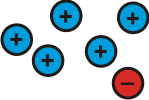and this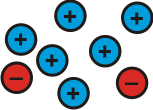and this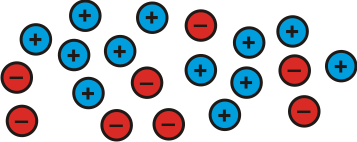Students could be asked to suggest some other possibilities.
Can they explain why they all represent $4$?

Let this be $^-2$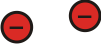and thisand this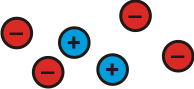and this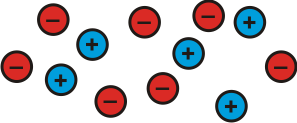Students could be asked to suggest some other possibilities.
Can they explain why they all represent $^-2$?

This is $3$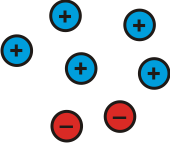When we partition it we can make different sums: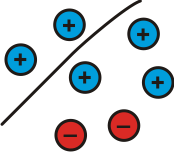$2 + 1 = 3$ $3 - 2 = 1$ $3 - 1 = 2$ Top + Bottom = Whole Whole - Top = Bottom Whole - Bottom = Top

If we partition it differently we can make different sums: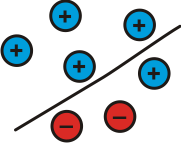$4 + (^-1) = 3$ $3 - 4 =$ $^-1$ $3 - (^-1) = 4$ Top + Bottom = Whole Whole - Top = Bottom Whole - Bottom = Top

And we can partition it differently again: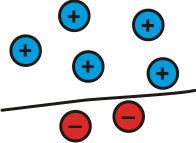$5 + (^-2) = 3$ $3 - 5 =$ $^-2$ $3 - (^-2) = 5$ Top + Bottom = Whole Whole - Top = Bottom Whole - Bottom = Top

This is $^-4$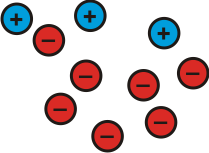When we partition it we can make different sums: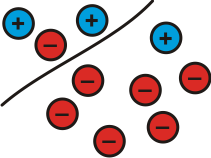$1 + (-5) =$ $^-4$ $^-4 - 1 =$ $^-5$ $^-4 - (^-5) = 1$ Top + Bottom = Whole Whole - Top = Bottom Whole - Bottom = Top

If we partition it differently we can make different sums: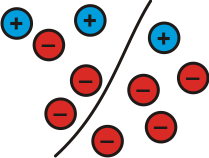$^-1 + (^-3) =$ $^-4$ $^-4 - (^-1) =$ $^-3$ $^-4 - (^-3) =$ $^-1$ Top + Bottom = Whole Whole - Top = Bottom Whole - Bottom = Top

Students could be asked to create three different sums based on their partitions of other sets of pluses and minuses.

How can this model help us make sense of adding negative numbers?

We can add a negative number to a positive number and end up with a positive solution:

 $6 + (^-2)$ = ?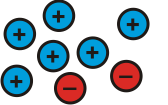=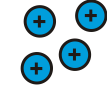$6 + (^-2)$ = 4

We can add a negative number to a positive number and end up with a negative solution:

 $4 + (^-6)$ = ?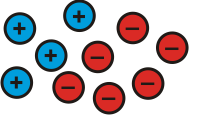=$4 + (^-6)$ = $^-2$

We can also add a negative number to a negative number:

 $^-2 + (^-3)$ = ?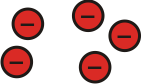=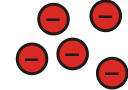$^-2 + (^-3)$ = $^-5$

Students could be asked to create a few more additions like the ones above.

Gathering various results may then offer an opportunity to ask students what they notice.
Can they suggest how to add negative numbers without going to all the trouble of drawing pluses and minuses?

How can this model help us make sense of subtracting negative numbers?

We can subtract a negative number from a negative number and end up with a negative solution:

Consider $^-8 - (^-3)$:

 This represents $^-8$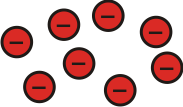And this represents $^-8$ subtract $^-3$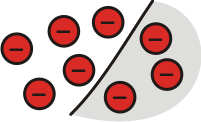Leaving us with $^-5$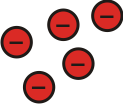So $^-8 - (^-3) =$ $^-5$

Can we subtract a negative number from a positive number?

Let's consider $5 - (^-2)$ = ?

How can we take $^-2$ from $5$?represents $5$

 but so does this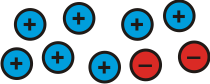Now we can take $^-2$ from $5$ and we will be left with: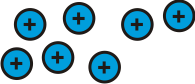So $5 - (^-2) = 7$

We can subtract a negative number from a negative number and end up with a positive solution!

Let's consider $^-4 - (^-7)$ = ?represents $^-4$

 but so does this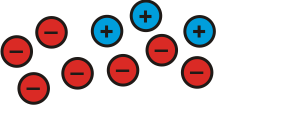Now we can take $^-7$ from $^-4$ and we will be left with: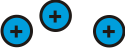So $^-4 - (^-7) = 3$

Students could be asked to create a few more subtractions like the ones above.

Gathering various results may then offer an opportunity to ask students what they notice.
Can they suggest how to subtract negative numbers without going to all the trouble of drawing pluses and minuses?

If you have a SMART Board, you may wish to download this notebook file which was shared with us by Jon Turpin.